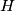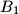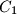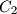### IMO Shortlist 2008 problem G1

Kvaliteta:
Avg: 3,0
Težina:
Avg: 6,0
Let$H$ be the orthocenter of an acute-angled triangle$ABC$. The circle$\Gamma_{A}$ centered at the midpoint of$BC$ and passing through$H$ intersects the sideline$BC$ at points$A_{1}$ and$A_{2}$. Similarly, define the points$B_{1}$,$B_{2}$,$C_{1}$ and$C_{2}$.

Prove that six points$A_{1}$ ,$A_{2}$,$B_{1}$,$B_{2}$,$C_{1}$ and$C_{2}$ are concyclic.

Author: Andrey Gavrilyuk, Russia
Izvor: Međunarodna matematička olimpijada, shortlist 2008